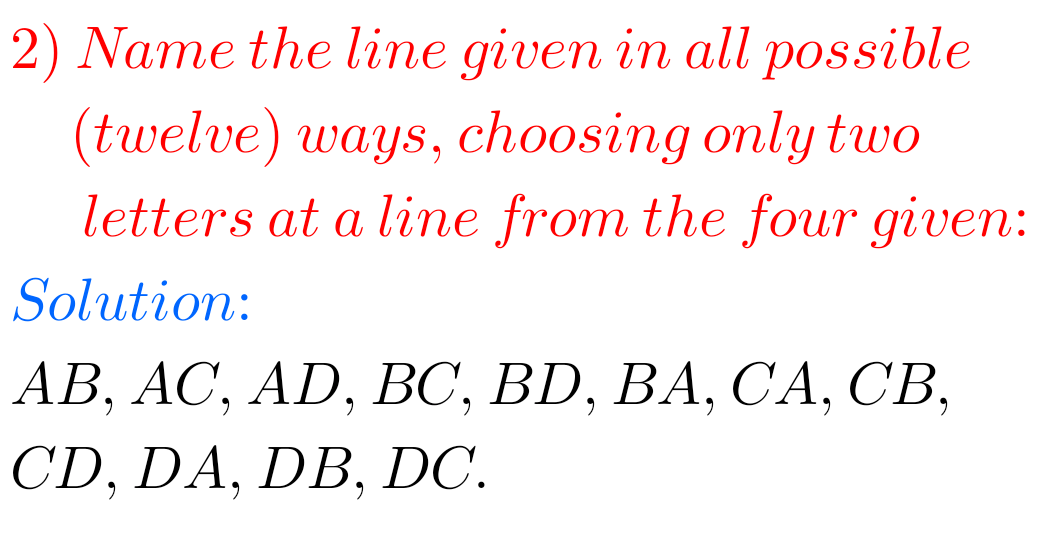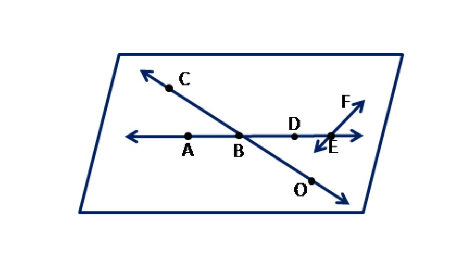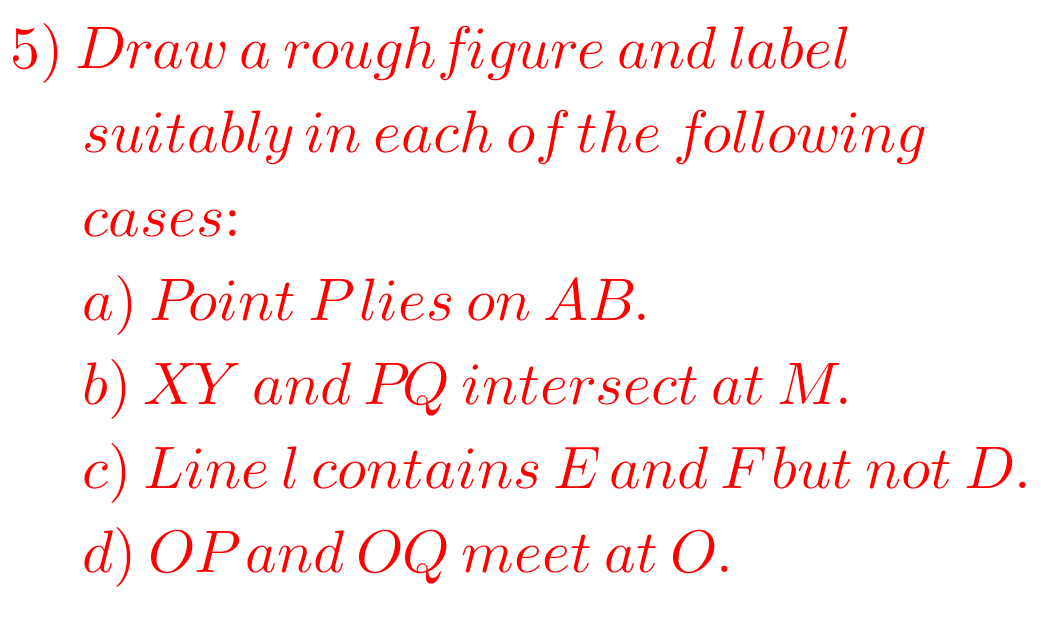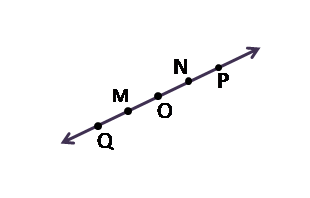# Basic Geometrical Ideas Solutions exercise 4.1 Class 6 Ncert Maths

Ncert textbook Mathematics Class 6 Chapter 4 Basic Geometrical Ideas exercise 4.1 solutions are given.

Study the lesson Basic Geometrical Ideas in the texbook.

Observe the problems and solutions given in the text book.

Observe the given below problems and solutions and try them in your own method.

You can see Solutions For Maths Class 6

Chapter 1 Knowing our Numbers

Chapter 2 whole Numbers

Some chapters links are given below

M

# Solutions for Basic Geometrical Ideas Class 6 Ncert Maths

Chapter 4 Basic Geometrical Ideas Exercise 4.1 Solutions

Exercise 4.1## Class 6 Maths Basic Geometrical Ideas Solutions exercise 4.1 Ncert Maths## Exercise 4.1 chapter 5 class 6 Ncert Maths Basic Geometrical IdeasM

Note : Observe the solutions and try them in your own method.

Chapter 3 Playing with Numbers

Chapter 4 Basic Geometrical Ideas

Exercise 4.1

Exercise 4.2

Exercise 4.3

Exercise 4.4

Exercise 4.5

Exercise 4.6

Chapter 6 Integers

Chapter 14 Practical Geometry

Exercise 14.1

Exercise 14.2

Exercise 14.3

Exercise 14.4

Exercise 14.5

Exercise 14.6

Fractions